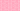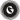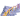PricingResources

# How to Calculate Balance Sheet RatiosWritten by

Calculating the ratios from a business’s balance sheet may seem like a dry and academic exercise, but it is incredibly valuable. If used correctly, a balance sheet equation enables the discovery of three vitally important metrics – the liquidity, solvency and profitability of a business.

• The liquidity refers to the ability to turn assets into cash and what this would produce.

• The solvency is a measure of the cash or equivalent assets available to pay debts.

• The profitability is the net profit the business is generating based on the balance sheet equation.

## How the balance sheet equation works

A range of different accounting formula is available for use, but they are all based on the three segments of any balance sheet, which are:

• Assets – the full value of the assets owned by the business

• Liabilities – the full value of the debts owed by the business

• Shareholders’ equity – the full value of the assets owned by the shareholders. Find out how to calculate shareholder's equity.

## How to use the balance sheet equation

Most of the information needed to create a balance sheet equation is available on the balance sheet itself, but sometimes the income sheet of a business may need to be consulted.

## The balance sheet equation and profitability

The profitability ratio is a measure of the money made by a business and how that money is divided to keep the business running and to reward investors.

## How do you calculate profitability ratios?

Profitability ratios show how much money a company makes and how it distributes the cash to operate and reward investors. The equation is broken down into these aspects:

• Gross profit – the amount left over after sales have been calculated, and all selling and administrative costs have been deducted.

• Contribution margin – the contribution margin measures what is left after all variable expenses are deducted from sales and shows the ratio of profit remaining to pay for fixed expenses.

• Net profit ratio – the ratio of the proceeds from sales left after the expenses are paid.

• Return on equity – the ratio of income to shareholders’ equity that shows investors what the return on their investment is.

• Return on assets – a measure of how much the assets of a business are effectively being used to drive profits for that business.

## How do you calculate liquidity ratios?

The liquidity ratio aspect of the accounting equation is a measure of the speed with which the business can pay off debts through cash reserves or liquified assets. The equation can be broken down as follows:

• Current ratio – the percentage of current assets over current liabilities. As a figure it is slightly hampered by it including inventory, which is difficult to turn into cash quickly.

• Quick ratiosimilar to the current ratio, but with the inventory subtracted.

• Cash ratio – compares the cash held and easily convertible investments to demonstrate how quickly debts could be paid with either or both.

## How do you calculate solvency ratios?

The solvency ratio aspect of an accounting equation demonstrates how effectively a business could pay off its debts. The current ratio and quick ratio outlined above could be used for both liquidity and solvency tests.

The lower a debt-to-equity ratio is, the better placed the business is. A debt of £10 and equity of £30 would deliver a debt-to-equity ratio of 0.333 using the accounting equation, and any figure less than one is generally judged to be a good sign of how the business is being run.

#### We can help

Calculating balance sheet ratios can feel like a complex part of running a business, and the same is true of taking payments from customers. Partnering with a payment platform like  GoCardless keeps this process as simple as possible and this includes the more complex aspects such as dealing with ad hoc payments or recurring payments.Contact sales

Sales

Support